Next: Particular forms of Up: Analysis of the Previous: Analysis of the

### Ambiguity

In eq:decomp,is uniquely determined. However,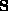is not. Indeed, ifsatisfies eq:decomp and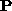is any invertible matrix which commutes with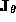, we have :So,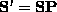satisfies eq:decomp too. The converse is also true. That is, if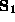and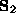both satisfy eq:decomp, then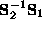is a matrix which commutes with. It can be easily shown that such a matrix can be written :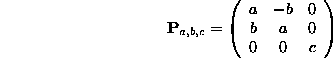Let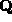be an orthogonal matrix such that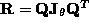. eq:ginf gives a real Jordan decomposition for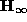: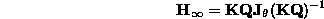Furthermore, ifhas been calculated by a real Jordan decomposition of(we will see later how to obtain it), we have, with respect to what has been shown previously, the following relationship : eq:ambiguity a,b,c =

Then,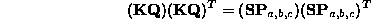And so, by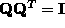,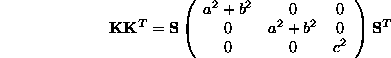Let be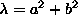and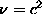. We have finally :

eq:kkt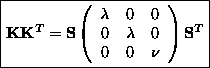Bob Fisher
Mon Dec 7 13:48:06 GMT 1998